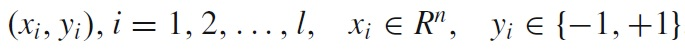SVM classifier is one of the latest and most successful supervised learning classifiers and has been widely applied for image classification due to its efficiency. Using training labeled samples, a statistical model is constructed and then new samples can be classified according to this trained model. The linear SVM aims at searching for an optimal hyperplane (or hyperplanes) in feature space with a large separating margin and a minimal misclassification rate.

For a binary linearSVMclassifier, given training data and its corresponding labels :the concrete formulation can be defined bywhere C > 0 is a penalty parameter to allow some misclassification and ξi are slack variables. The objective function aims to maximize the margin and the constraints indicate that the training points should be correctly classified by the relaxed decision function wT x +b. To extend binary SVM for multi-class problems, we use the one-vs-all approach . We train a single binary SVM classifier per class by treating the cell images of this class as positive samples and those of other classes as negative samples. When classification is performed, all the binary classifiers are run and the classifier with the highest confidence score is chosen.

MATLAB introduce new function for SVM in MATLAB2014a.

Last function has some disadvantage , but new function was better than last function.

fitcsvm

is function for Train binary support vector machine classifier.

`crossval`

Cross-validate the classifier using 10-fold cross-validation